Question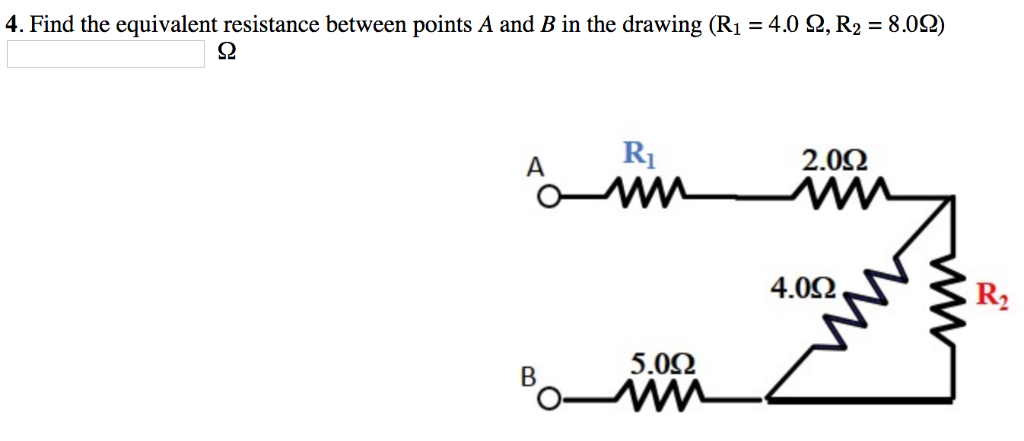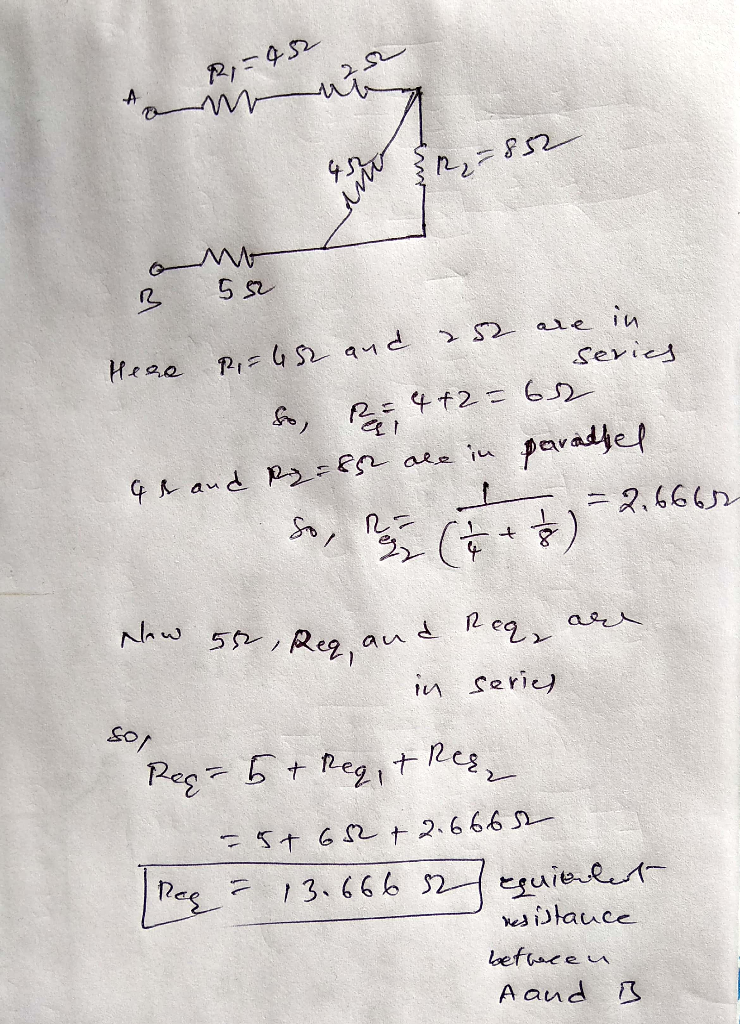#### Earn Coins

Coins can be redeemed for fabulous gifts.

Similar Homework Help Questions
• ### Find the equivalent resistance between points A and B in the drawing. 3.0 Ω 4.0 2...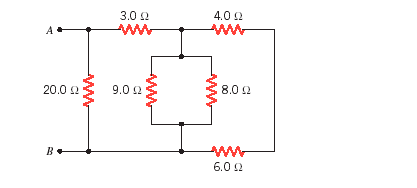Find the equivalent resistance between points A and B in the drawing. 3.0 Ω 4.0 2 8.0 Ω 20012 9.0 12 6.0 Ω

• ### Find the equivalent resistance between points A and B in the drawing. Find the equivalent resistance...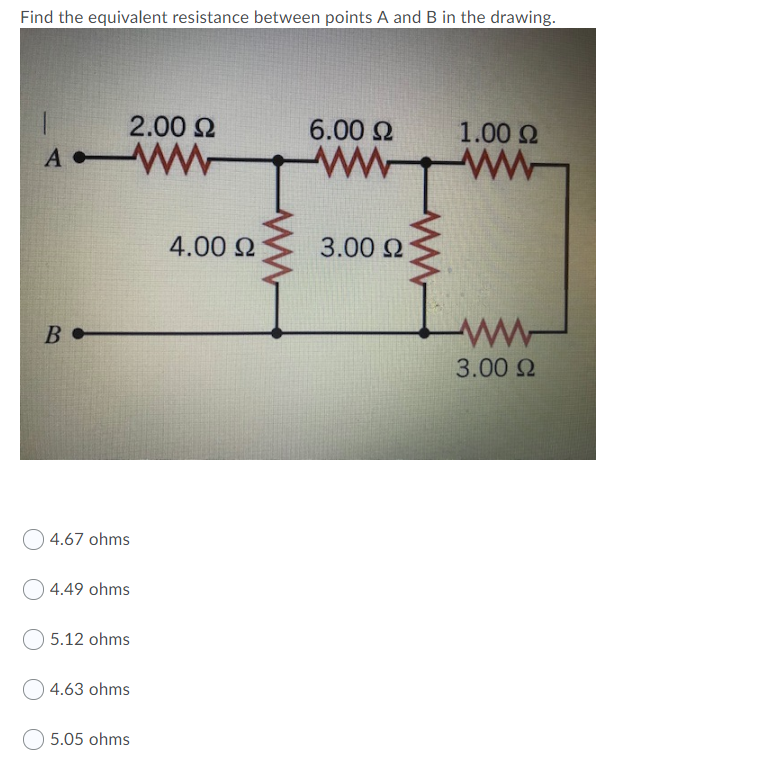Find the equivalent resistance between points A and B in the drawing. Find the equivalent resistance between points A and B in the drawing. 2.00 Ω 6.00 Ω 1.00 Ω A 4.00 Ω 3.00 Ω B - ΑΛΛ- 3.00 Ω 4.67 ohms Ο4.49 ohms 5.12 ohms 4.63 ohms 5.05 ohms

• ### Find the equivalent resistance between points A and B in the drawing. 3.0 4.0 A, a...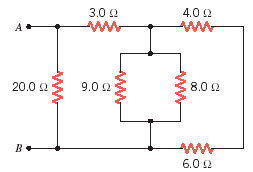Find the equivalent resistance between points A and B in the drawing. 3.0 4.0 A, a WW WW 20.0 9.0 2 8.0 6.0

• ### Find the equivalent resistance between points A and B in the drawing. RI 26 Ω R2...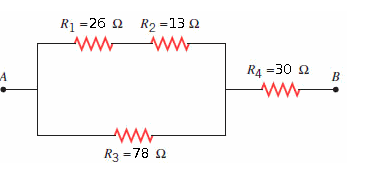Find the equivalent resistance between points A and B in the drawing. RI 26 Ω R2 1362 R4-30 Ω Ra 78 Ω

• ### 9. Determine the equivalent resistance between the points A and B for the group of resistors...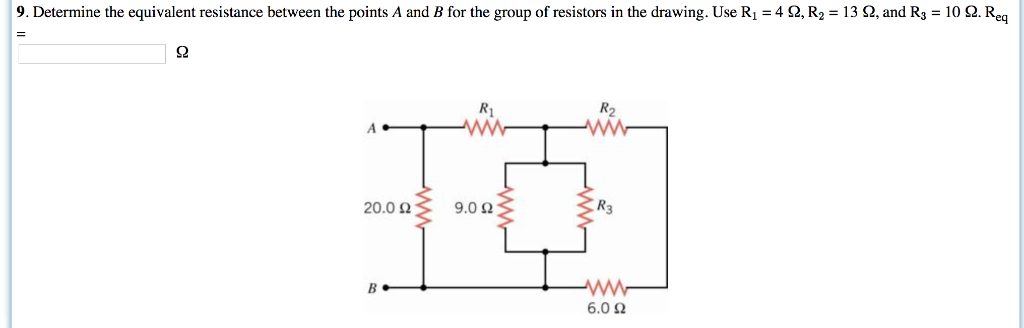9. Determine the equivalent resistance between the points A and B for the group of resistors in the drawing. Use R1-4 Ω, R2 = 13 Ω and R3 = 10 Ω Re Ri R2 20.0 Ω 9.0 Ω R3 6.0 Ω

• ### Find the equivalent resistance between points A and B shown in the figure(Figure 1). Consider R1...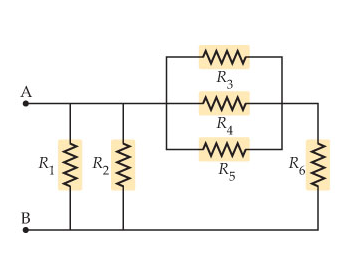Find the equivalent resistance between points A and B shown in the figure(Figure 1). Consider R1 = 2.6 Ω , R2 = 3.7 Ω , R3 = 4.1 Ω , R4 = 4.3 Ω , R5 = 6.1 Ω , and R6 = 6.2 Ω . w MM

• ### Find the equivalent resistance between points A and B shown in the figure(Figure 1). Consider R1...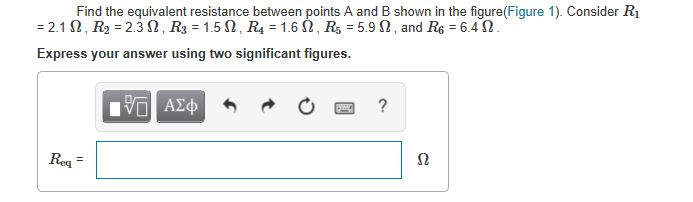Find the equivalent resistance between points A and B shown in the figure(Figure 1). Consider R1 -2.1 Ω , R2 = 2.3 Ω . R3 = 1.5 Ω , R4 = 1.6 Ω . R5 = 5.9 Ω . and R6 = 6.462 Express your answer using two significant figures. Req -

• ### QUESTION 1 3 points Save Answer Find the equivalent resistance between terminals A and B. R1...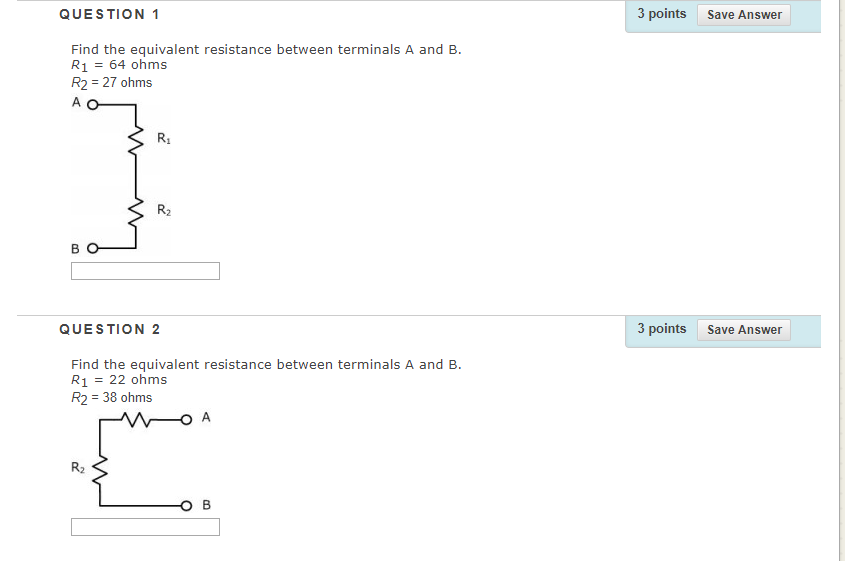QUESTION 1 3 points Save Answer Find the equivalent resistance between terminals A and B. R1 64 ohms R2 27 ohms R1 R2 QUESTION 2 3 points Save Answer Find the equivalent resistance between terminals A and B. R1 = 22 ohms R2 38 ohms R2

• ### Find the equivalent resistance between points A and B in the drawing 2.00 Ω 6.00 Ω...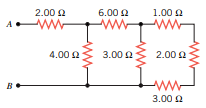Find the equivalent resistance between points A and B in the drawing 2.00 Ω 6.00 Ω 1.00 Ω wongan 4.00 Ω 3.00Ω 2.00 Ω B 3.00 Ω

• ### Situation #2: Consider the circuit shown below: V1 = 4.0 V R1 = 8.0 Ω R2...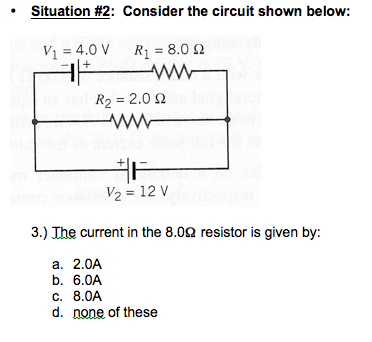Situation #2: Consider the circuit shown below: V1 = 4.0 V R1 = 8.0 Ω R2 = 2.0Ω V2 = 12V 3) The current in the 8.0Ω resistor is given by: a. 2.0A b. 6.0A c. 8.0A d. none of these